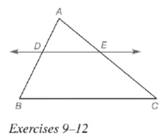Chapter 5.6, Problem 10EElementary Geometry For College St...

7th Edition
Alexander + 2 others
ISBN: 9781337614085

Solutions

Chapter
SectionElementary Geometry For College St...

7th Edition
Alexander + 2 others
ISBN: 9781337614085
Textbook Problem

Given: D E ↔ ∥ B C , ¯ A D = 6 , D B = 10 , A C = 20 Find: E CTo determine

To find:

EC.

Explanation

Given:

Theorem used:

If a line is parallel to one side of a triangle and intersects other two sides, then it divides these sides proportionally.

Formula used:

If ab=cd then a+bb=c+dd.

Calculation:

Given: DEBC¯

Since if a line is parallel to one side of a triangle and intersects other two sides, then it divides these sides proportionally.

We know that, if ab=cd then a+bb=c+dd.

From the figure, we see that AE+EC=AC

Still sussing out bartleby?

Check out a sample textbook solution.

See a sample solution

The Solution to Your Study Problems

Bartleby provides explanations to thousands of textbook problems written by our experts, many with advanced degrees!

Get Started

Find more solutions based on key concepts